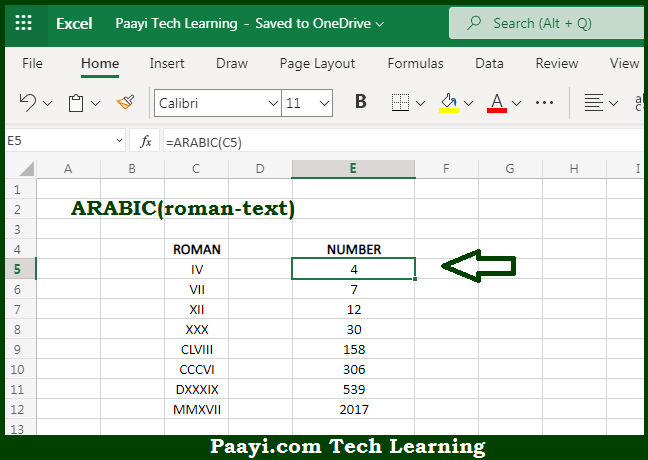# Learn How to Use Microsoft Excel ARABIC Function

Written by | 0 Comments | 609 Views

In this article, you will learn how to use the Microsoft Excel ARABIC function and its prime function in Microsoft Excel. You will also get to know the Microsoft Excel ARABIC function return value and syntax with the help of some examples.

Microsoft Excel ARABIC Function

The main function of the Microsoft Excel ARABIC function is to convert roman numerals to Arabic numerals. So, with the help of the Arabic function, you can able to convert the roman numerals to the Arabic numerals in the form of a text or to a number in an Arabic numeral. So, with the help of the Microsoft Excel ARABIC function, you can easily return the ARABIC numerals.

Return Value of ARABIC Function

The return value will be the number in the Arabic numeral.

Syntax of ARABIC Function

=ARABIC(roman-text)

Where the arguments:

roman-text: This is the Roman numeral in the text which you want to convert.

How to Use Microsoft Excel ARABIC Function?Microsoft Excel ARABIC function is used to convert the Roman numeral into the Arabic numeral. It should be noted that the maximum length of the "roman-text" is 255 characters and the Arabic function just do the opposite of the Roman numeral. So, with the help of the Microsoft Excel ARABIC function, you can easily return the ARABIC numerals.